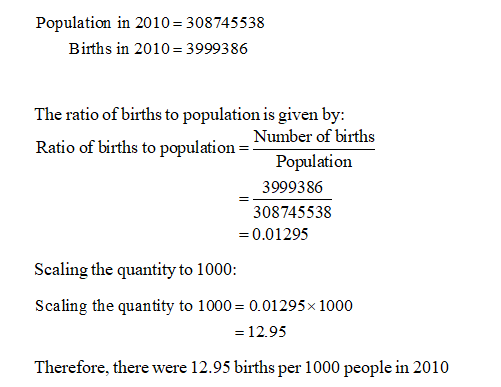# The US Census in 2010 counted 308,745,538 people and there were 3,999,386 births that year.1.) compute the ratio of births to population and scale the second quantity to 1,000. 2.) This rate is 3% lower than the 2009 birth rate. Give one possible explanation why the rate is lower given that the population grew.

Question
1 views

The US Census in 2010 counted 308,745,538 people and there were 3,999,386 births that year.

1.) compute the ratio of births to population and scale the second quantity to 1,000.

2.) This rate is 3% lower than the 2009 birth rate. Give one possible explanation why the rate is lower given that the population grew.

check_circle

Step 1

Given:...

### Want to see the full answer?

See Solution

#### Want to see this answer and more?

Solutions are written by subject experts who are available 24/7. Questions are typically answered within 1 hour.*

See Solution
*Response times may vary by subject and question.
Tagged in

### Statistics